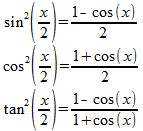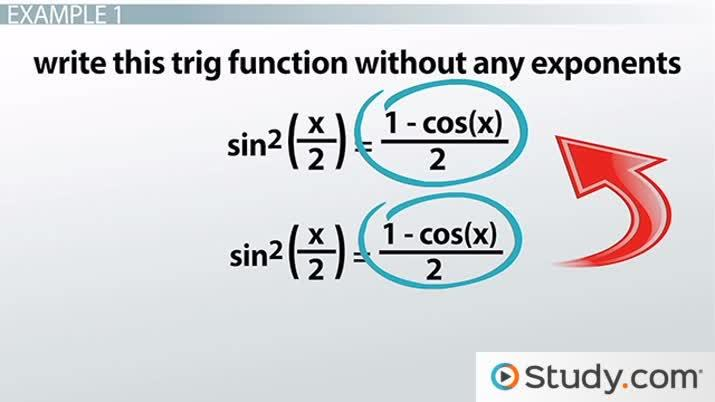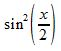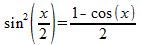Half-Angle Identities: Uses & Applications

Lesson Transcript
Instructor: Yuanxin (Amy) Yang Alcocer

Amy has a master's degree in secondary education and has been teaching math for over 9 years. Amy has worked with students at all levels from those with special needs to those that are gifted.

Half-angle identities are simply true statements about half-angles that can simplify certain trigonometry problems. Learn the ways these identities are used and applied through two example scenarios provided. Updated: 10/20/2021

Half-Angle Identities

In this video lesson, we are covering the half-angle identities of trigonometry, which are the true statements for half-angles. These are definitions, if you will. In other words, they tell you what a particular trig function equals. You can see here that they take a squared trig function and turn it into a trig function without exponents:We have one half-angle for each of our three basic trig functions. We have one for sine, one for cosine, and one for tangent. As you can see, our angle has been halved, hence the name half-angle identity. On the left side, our trig function is squared, and on the right, we see the equivalent statement in terms of cosine without any exponents. Also, the angle on the right side is no longer halved.An error occurred trying to load this video.

Try refreshing the page, or contact customer support.

Coming up next: Product-to-Sum Identities: Uses & Applications

You're on a roll. Keep up the good work!

Replay
Your next lesson will play in 10 seconds
• 0:01 Half-Angle Identities
• 0:42 Uses and Applications
• 1:30 Example 1
• 2:10 Example 2
• 3:07 Lesson Summary
Save Save

Want to watch this again later?

Timeline
Autoplay
Autoplay
Speed Speed

Uses and Applications

So, what can you do with these identities? We use these identities when we need help simplifying a trig function. When we have a squared trig function, it is sometimes difficult to work with in higher math. So, if we turn it into an equivalent statement without exponents, then it will help us solve the problem that much more easily.

You can think of these half-angle trig identities as a key that helps you to decode or simplify a harder problem. Without this key, you might not be able to solve the problem at all. But with the key, you are able to find your way to the answer. Also, these identities are used to prove yet other trig identities or statements. Let's look at a couple of examples now to see what we can do with these trig identities.

Example 1

This problem is asking us to write this trig function without any exponents:At first glance, we might think that this problem is already as simple as it can get. It only has one function: the sine function. But the problem wants us to write it so that we don't have the square. How can we do that?

Well, we look at the angle and notice that our angle is being halved. Ah. We can see if we can use one of our half-angle identities. We look at our list. Oh, look! Here, we have a sine squared function that is equal to something without any exponents. We can use that one to get our answer:And there we have our answer without any exponents. This problem was a pretty direct problem with a simple direct answer. Let's look at another problem.

To unlock this lesson you must be a Study.com Member.

Register to view this lesson

Are you a student or a teacher?